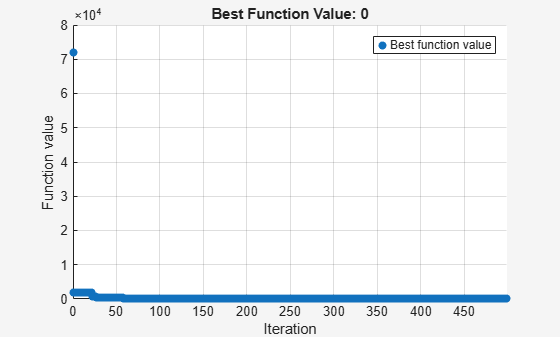# Mixed-Integer Surrogate Optimization

This example shows how to solve an optimization problem that involves integer variables. Beginning in R2019b, `surrogateopt` accepts integer constraints. In this example, find the point `x` that minimizes the `multirosenbrock` function over integer-valued arguments ranging from –3 to 6 in ten dimensions. The `multirosenbrock` function is a poorly scaled function that is difficult to optimize. Its minimum value is 0, which is attained at the point `[1,1,...,1]`.

```rng(1,'twister') % For reproducibility nvar = 10; % Any even number lb = -3*ones(1,nvar); ub = 6*ones(1,nvar); fun = @multirosenbrock; intcon = 1:nvar; % All integer variables [sol,fval] = surrogateopt(fun,lb,ub,intcon)``````Surrogateopt stopped because it exceeded the function evaluation limit set by 'options.MaxFunctionEvaluations'. ```
```sol = 1×10 1 1 0 0 0 0 1 1 -1 1 ```
```fval = 6 ```

In this case, `surrogateopt` does not find the correct solution.